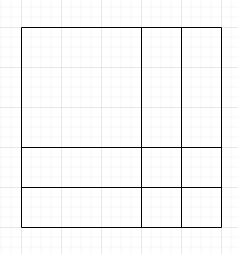# Understanding ROI Pool of fast r-cnn

Hello all,

I am facing difficulty when understanding the roi pool layer that was implemented in torchvision `torchvision.ops.roi_pool`. I have attached the sample code below

``````import torch
import torchvision

import numpy as np
feature_map = np.array([
[0.70, 0.41, 0.38, 1.23, 0.24],
[0.14, 0.45, 0.31, 0.73, 3.22],
[0.11, 0.41, 0.79, 0.69, 0.44],
[1.47, 0.25, 0.09, 0.32, 2.98],
[0.48, 0.87, 0.77, 0.26, 0.11],
])

feature_map = torch.tensor(feature_map, requires_grad=False).float()

# (batch, channel, h, w) -> (1, 1, 5, 5)
feature_map = feature_map.unsqueeze(0).unsqueeze(0)

# boxes -> (1, 5)
boxes = np.array([
[0, 0, 0, 4, 4],
])
boxes = torch.tensor(boxes, requires_grad=False).float()

# roi pooling layer of 3x3
pool = torchvision.ops.roi_pool(input=feature_map, boxes=boxes, output_size=3)
print(pool)
``````

The output of the above code is

``````tensor([[[[0.7000, 1.2300, 3.2200],
[1.4700, 0.7900, 3.2200],
[1.4700, 0.8700, 2.9800]]]])
``````

So here the `5x5` map is divided in such a way that the output is `3x3`. So `5x5` region can be divided the following way to `3x3` (there are many ways but I only drew 2)• How the subregions are decided in pytorch code (I checked the paper and there was no detail on this one). Is there any algorithm pytorch is referring to ?
• My understanding says that there should be no overlap between sub-regions but that doesn’t explain the output of above code (as you can see `3.22` is present in (0, 2) and (1, 2) positions of output)

The versions I am using are :

``````torch = 1.10.2+cpu
torchvision = 0.11.3+cpu
``````

Thanks.

1 Like